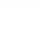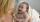# Mr and Mrs

Mr. Calda and Mrs. Cald have a total of 139 years. How many years have when we know that Mr. Calda is 9 years older than Mrs. Cald

Result

a =  74
b =  65

#### Solution:

a+b = 139
a = 9 + b

a+b = 139
a-b = 9

a = 74
b = 65

Calculated by our linear equations calculator.

Leave us a comment of example and its solution (i.e. if it is still somewhat unclear...):

Showing 0 comments:Be the first to comment!#### To solve this example are needed these knowledge from mathematics:

Do you have a system of equations and looking for calculator system of linear equations?

## Next similar examples:

1. Three brothersThe three brothers have a total of 42 years. Jan is five years younger than Peter and Peter is 2 years younger than Michael. How many years has each of them?
2. Mother and daughterThe mother is four times older than her daughter. Five years ago, her daughter was seven times younger than her mother. How many years do they have now?
3. Two numbersWe have two numbers. Their sum is 140. One-fifth of the first number is equal to half the second number. Determine those unknown numbers.
4. Adam and BenWhen Ben is so many years old to Adam today, Adam will be 23 years old. When Adam was as old as Ben, Ben was two years old. How old is today Ben and Adam?
5. Mother and daughter 2The mother is 40 years older than her daughter. How old is the mother if her age is eight thirds age of daughter?
6. Ages 2A man's age is 4 times his son's age. After 5 years he will be just twice his son's age, find their ages.
7. YearsSusan is 3 years and as she will be old as Teodor now him will be 11. How old is Teodor today?
8. Three daysDuring the three days sold in stationery 1490 workbooks. The first day sold about workbooks more than third day. The second day 190 workbooks sold less than third day. How many workbooks sold during each day?
9. TheatroTheatrical performance was attended by 480 spectators. Women were in the audience 40 more than men and children 60 less than half of adult spectators. How many men, women and children attended a theater performance?
10. Three friendsThe three friends spent 600 KC in a teahouse. Thomas paid twice as much as Paul. Paul a half less than Zdeněk. How many each paid?
11. Jane plantsJane plants flowers in the garden. If she planted 12 every hour instead of 9 flowers, she would finish with the job an hour earlier. How many flowers does she plant?
12. MushroomsEva and Jane collected 114 mushrooms together. Eve found twice as much as Jane. How many mushrooms found each of them?
13. TreesAlong the road were planted 250 trees of two types. Cherry for 60 CZK apiece and apple 50 CZK apiece. The entire plantation cost 12,800 CZK. How many was cherries and apples?
14. Hotel roomsIn the 45 rooms, there were 169 guests, some rooms were three-bedrooms and some five-bedrooms. How many rooms were?
15. Equations - simpleSolve system of linear equations: x-2y=6 3x+2y=4
16. Linear systemSolve this linear system (two linear equations with two unknowns): x+y =36 19x+22y=720
17. EquationsSolve following system of equations: 6(x+7)+4(y-5)=12 2(x+y)-3(-2x+4y)=-44